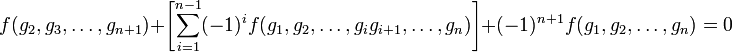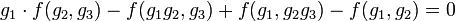# Cocycle for a group action

## Definition

Suppose$G$ is a group and$A$ is an abelian group, with an action$\varphi$ of$G$ on$A$. In other words,$\varphi$ is a homomorphism of groups from$G$ to$\operatorname{Aut}(A)$, the automorphism group of$A$.

### Definition in terms of bar resolution

A$n$-cocycle is an element in the$n^{th}$ cocycle group for the Hom complex from the bar resolution of$G$ to$A$, in the sense of$\mathbb{Z}G$-modules.

### Explicit definition

For$n$ a nonnegative integer, a$n$-cocycle for the action$\varphi$ of$G$ on$A$ is a function$f:G^n \to A$ such that, for all$g_1,g_2, \dots, g_{n+1} \in G$:$\! \varphi(g_1)(f(g_2,g_3,\dots,g_{n+1})) + \left[ \sum_{i=1}^{n-1} (-1)^i f(g_1,g_2, \dots,g_ig_{i+1},\dots,g_n)\right] + (-1)^{n + 1}f(g_1,g_2,\dots,g_n) = 0$

If we suppress the symbol$\varphi$ and denote the action by$\cdot$, this becomes:$\! g_1 \cdot f(g_2,g_3,\dots,g_{n+1})) + \left[ \sum_{i=1}^{n-1} (-1)^i f(g_1,g_2, \dots,g_ig_{i+1},\dots,g_n)\right] + (-1)^{n+1} f(g_1,g_2,\dots,g_n) = 0$

In particular, when the action is trivial, this is equivalent to saying that:$\! f(g_2,g_3,\dots,g_{n+1}) + \left[ \sum_{i=1}^{n-1} (-1)^i f(g_1,g_2, \dots,g_ig_{i+1},\dots,g_n)\right] + (-1)^{n+1} f(g_1,g_2,\dots,g_n) = 0$

## Particular cases$n$ Condition for being a$n$-cocycle Further information
1 For all$g_1,g_2\in G$, we have$\! g_1 \cdot f(g_2) - f(g_1g_2) + f(g_1) = 0$, equivalently$\! f(g_1g_2) = f(g_1) + g_1 \cdot f(g_2)$ 1-cocycle for a group action
2 For all$g_1,g_2,g_3\in G$, we have$\! g_1 \cdot f(g_2,g_3) - f(g_1g_2,g_3) + f(g_1,g_2g_3) - f(g_1,g_2) = 0$, equivalently$g_1 \cdot f(g_2,g_3) + f(g_1,g_2g_3) = f(g_1g_2,g_3) + f(g_2,g_3)$ 2-cocycle for a group action
3 For all$g_1,g_2,g_3,g_4 \in G$, we have$\!g_1 \cdot f(g_2,g_3,g_4) - f(g_1g_2,g_3,g_4) + f(g_1,g_2g_3,g_4) - f(g_1,g_2,g_3g_4) + f(g_1,g_2,g_3) = 0$, or equivalently,$\! g_1 \cdot f(g_2,g_3,g_4) + f(g_1,g_2g_3,g_4) + f(g_1,g_2,g_3) = f(g_1g_2,g_3,g_4) + f(g_1,g_2,g_3g_4)$ 3-cocycle for a group action